Shared Concepts and Topics

### Truncated Power Function Basis

A truncated power function for a knot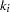is a function defined by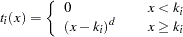Figure 18.1 shows such functions for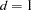and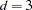with a knot at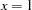.

Figure 18.1 Truncated Power Functions with Knot at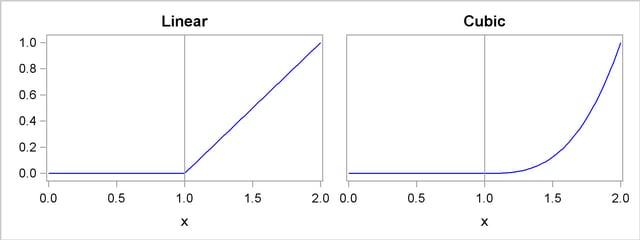The name is derived from the fact that these functions are shifted power functions that get truncated to zero to the left of the knot. These functions are piecewise polynomial functions with two pieces whose function values and derivatives of all orders up to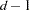are zero at the defining knot. Hence these functions are splines of degree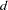. It is easy to see that thesefunctions are linearly independent. However, they do not form a basis, because such a basis requires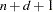functions. The usual way to add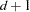additional basis functions is to use the polynomials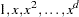. Thesefunctions together with thetruncated power functions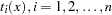form the truncated power basis.

Note that each time a knot is repeated, the associated exponent used in the corresponding basis function is reduced by 1. For example, for splines of degreewith 3 repeated knots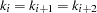the corresponding basis functions are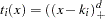,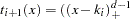, and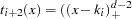. Provided that the multiplicity of each repeated knot is less than or equal to the degree, this construction continues to yield a basis for the associated space of splines.

The main advantage of the truncated power function basis is the simplicity of its construction and the ease of interpreting the parameters in a model corresponding to these basis functions. However, there are two weaknesses when you use this basis for regression. These functions grow rapidly without bound asincreases, resulting in numerical precision problems when thedata span a wide range. Furthermore, many or even all of these basis functions can be nonzero when evaluated at somevalue, resulting in a design matrix with few zeros that precludes the use of sparse matrix technology to speed up computation. This weakness can be addressed by using a B-spline basis.Previous Page | Next Page | Top of Page